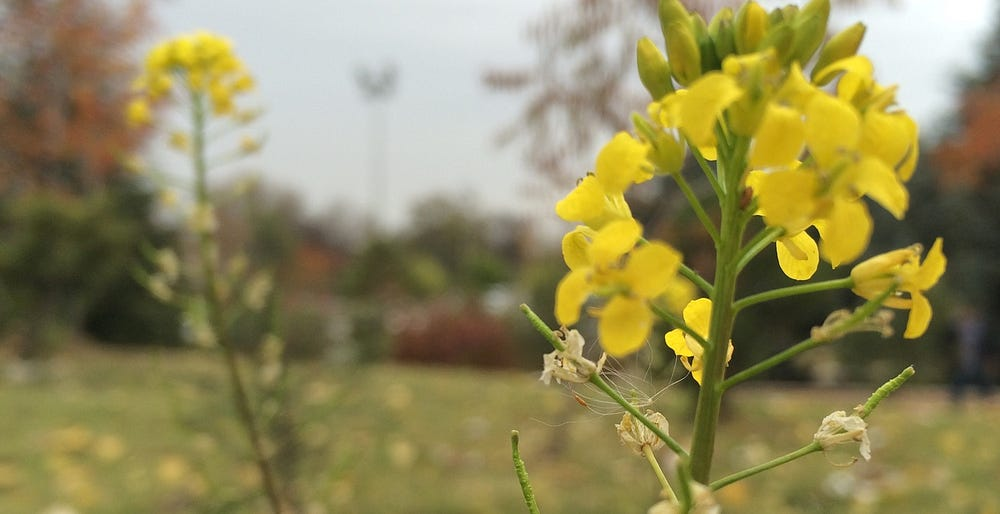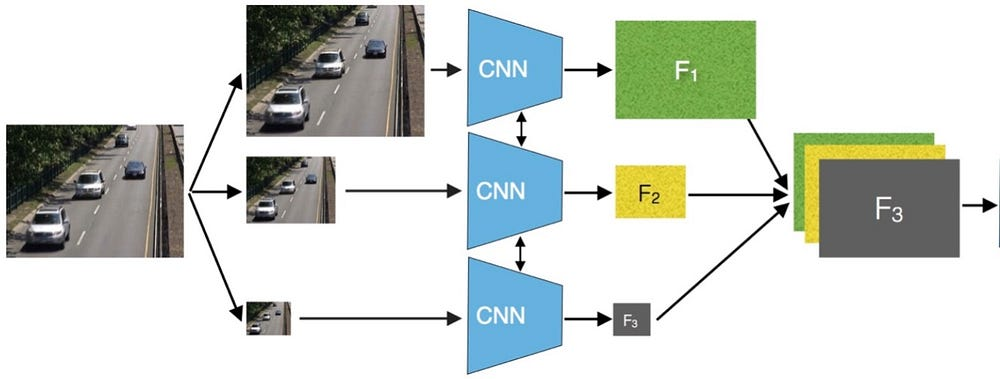# Tips for Keras deep learning framework’s fans

Source: Deep Learning on Medium

In this article, I’m going to give you a few useful tips when working with Keras framework. Now, let’s get started…a moment in AU campus

#### 1. How to deploy small sized-keras model to the web or mobile?

When deploying deep learning models, especially Keras models, to a free web hosting (e.g. Heroku), it’s important to make your trained models small as much as possible since the web hosting server limited memory size for each deployed application. Normally, after training a Keras model, you would save and load the model like this:

`# during trainingmodel.fit(...)`
`model.save(‘mdl.h5’) #e.g. mdl.h5 size = 38.3MB on disk`
``# `load model back on the web/mobile`from keras.models import load_model`model = load_model(‘mdl.h5’)`

However, `model.save('...')` will save all model configurations (see this for details). Instead of deploying a large sized-model (with all configurations), you would save/load only model’s architecture and weights, like this:

`# during trainingmodel.fit(...)`
`with open(‘mdl.json’, ‘w’) as f:    f.write(model.to_json())    #e.g. mdl.json size = 45KB on disk`
`model.save_weights(‘weights.h5’) #e.g. weights.h5 size = 17.3MB`
``# `load model back on the web/mobilefrom keras.models import model_from_jsonwith open('mdl.json','r') as f:     json = f.read()model = model_from_json(json)model.load_weights('weights.h5')`

As can be seen, the server needs to load 38.3MB sized-model by using the first approach. However, it needs to load only 17.3MB+45KB ~17.34MB by using the second approach, which reduces the model size about 20.96MB !!! Cool!

#### 2. How to remove/replace the activation function?

Assume that you have a pre-trained network where the layer that you want to remove/replace the activation function is built using the following form:

`x = Conv2D(num_features, kernel_size, activation=’relu’, name='conv_1')(x)`
• If you want to remove the activation:
`from keras.models import load_modelfrom keras.layers import Activationfrom keras.utils.generic_utils import get_custom_objects`
`# create a fake activation which returns the raw featuresdef fake_activation(x):return x`
`# load the pre-trained modelmodel = load_model('pre-trained.h5')`
`# remove the activation from layer_namelayer_name = 'conv_1'model.get_layer(layer_name).activation = fake_activation`
`# save the modelmodel.save('my_replace.h5')`
`# and reload it backmodel = load_model('my_replace.h5')`
• If you want to replace the layer with another activation:
`# ... the rest of codes are same as above`
`def custom_activation(x):# ... your custom code here. e.g. softmax function:     #     x = keras.activations.softmax(x, axis=-1)return x`
`layer_name = 'conv_1'model.get_layer(layer_name).activation = custom_activation`
`# ... the rest of codes are same as above`

Benefit: It allows you to tweak the activation function in the network to seeing how the model performs when changing the activation function.

#### 3. How to train a multi-inputs and multi-outputs model?

Suppose that you have a model which consists of two inputs and two outputs. Keras has capability to do so:

`from keras.models import Modelfrom keras.layers import Input, add, Densefrom keras.layers.convolutional import Conv2D`
`#first inputinput_1 = Input(shape=(20,30,3))x1 = Conv2D(64, (3,3), activation='relu')(input_1)x1 = Conv2D(64, (3,3), activation='relu')(x1)`
`#second inputinput_2 = Input(shape=(20,30,3))x2 = Conv2D(64, (3,3), activation='relu', dilation_rate=2)(input_2)x2 = Conv2D(64, (3,3), activation='relu', dilation_rate=4)(x2)`
`x = add([x1,x2])x = Conv2D(128, (3,3), activation='relu')(x)x = Conv2D(128, (3,3), activation='relu')(x)`
`#two outputs c1, c2c1 = Conv2D(1, (1,1), activation='sigmoid')(x)c2 = Dense(4, activation='softmax')(x)`
`model = Model(inputs=[input_1, input_2], outpus=[c1, c2])model.compile(...)`

#### 4. How to share weights between two or more models?

Sharing weights is widely used in practice. Usually, when we fit two or more models with different scaled images. Assume that you feed three different scaled images to three CNN models, where their weights are shared. Then, the three output features are concatenated along the depth axis. Finally, the concatenated features are past to the next layer (could be a classifier):Source from here

You could build the network in Keras like this:

`from keras.models import Modelfrom keras.layers import Input, concatenatefrom keras.layers.convolutional import Conv2D, UpSampling2D`
`w = h = 224 #image width/heightd = 3 #image channel`
`#scale 1input_1 = Input(shape=(h, w, d))`
`x = Conv2D(64, (3,3), activation='relu')(input_1)x = Conv2D(128, (3,3), activation='relu')(x)`
`#create a shared modelshared_model = Model(inputs=input_1, outputs=x) x1 = shared_model.output`
`#scale 2input_2 = Input(shape=(int(h/2), int(w/2), d))x2 = shared_model(input_2)x2 = UpSampling2D((2,2))(x2)`
`#scale 3input_3 = Input(shape=(int(h/4), int(w/4), d))x3 = shared_model(input_3)x3 = UpSampling2D((4,4))(x3)`
`#concatenate the three outputs (tf backend)top = concatenate([x1, x2, x3], axis=-1)`
`#here you can pass <top> output features to the next layer!!!`

These are some tips!!! I will add more tips and tricks to this post if any.

### Final words…

If you like this post, feel free to clap or share it. If you have any questions, please drop in comments below. You can connect me on LinkedIn, or follow me here. Have a nice day!